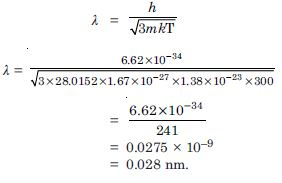# NCERT Solutions for Class 12 Physics Chapter 1 - Dual Nature of Matter And Radiation

Aasoka provides important reference material for students of Class 12 which includes NCERT Solutions as per the updated syllabus. This provides students with the perfect educational resources to start their exam preparation in order to score good marks. NCERT Solutions for Class 12th is designed in accordance with the students’ educational requirements which makes it easier for them to kickstart their preparation for board exams. Students can access free solutions on the Aasoka website in order to boost their performance.

“Dual Nature of Matter and Radiation” chapter includes topics such as Einstein’s Photoelectric equation, electron emission, experimental effect, the wave nature of matter, photoelectric effect, wave theory, and so much more. Along with this, students will learn whether radiation is similar to a particle or not.

##### Question 1:

Find the
(a) maximum frequency and
(b) minimum wavelength of X-rays produced by 30 kV electrons.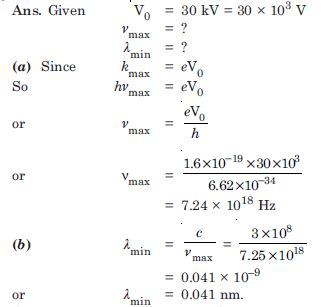##### Question 2:

The work function of caesium metal is 2.14 eV. When light of frequency 6 × 1014 Hz is incident on the metal surface, photoemission of electrons occurs. What is the maximum kinetic energy of the emitted electrons, stopping potential and maximum speed of the emitted photoelectrons ?

Given W0 = 2.14 eV
= 2.14 × 1.6 × 10 –19 J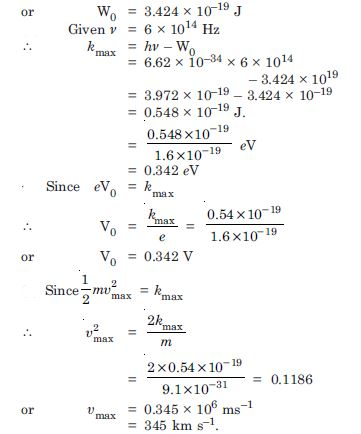##### Question 3:

The photoelectric cut-off voltage in a certain experiment is 1.5 V. What is the maximum kinetic energy of photoelectrons emitted ?##### Question 4:

Monochromatic light of wavelength 632.8 nm is produced by a helium-neon laser.
The power emitted is 9.42 mW.
Find the energy and momentum of each photon in the light beam.
How many photons per second, on the average, arrive at a target irradiated by this beam ? (Assume the beam to have uniform crosssection which is less than the target area), and How fast does a hydrogen atom have to travel in order to have the same momentum as that of the photon ?

Given $\mathrm{\lambda }$ = 632.8 nm
= 632.8 × 10–9 m
P = 9.42 mW = 9.42 × 10–3 W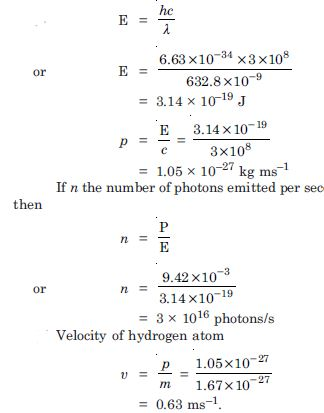##### Question 5:

The energy flux of sunlight reaching the surface of the earth is 1.388 × 103 W/m 2. How many photons (nearly) per square metre are incident on the Earth per second ? Assume that the photons in the sunlight have an average wavelength of 550 nm.

Energy flux = 1.388 × 103 W/m2
$\mathrm{\lambda }$ = 550 nm = 550 × 10–9 m
$\therefore$ number of photons per square metre incident per second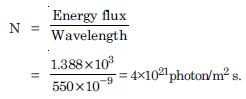##### Question 6:

A 100 W sodium lamp radiates energy uniformly in all directions. The lamp is located at the centre of a large sphere that absorbs all the sodium light which is incident on it. The wavelength of the sodium light is 589 nm. (a) What is the energy per photon associated with the sodium light ? (b) At what rate are the photons delivered to the sphere

Given P = 100 W
$\mathrm{\lambda }$ = 589 nm
= 589 × 10–9 m
Energy per photon,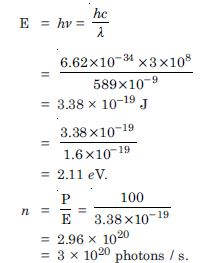##### Question 7:

The threshold frequency for a certain metal is 3.3 × 1014. Hz. If light of frequency 8.2 × 1014 Hz is incident on the metal, predict the cutoff voltage for the photoelectric emission.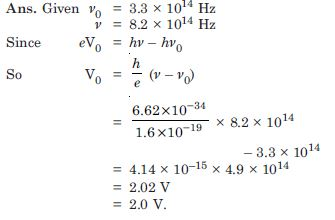##### Question 8:

The work function for a certain metal is 4.2 eV. Will this metal give photoelectric emission for incident radiation of wavelength 330 nm ?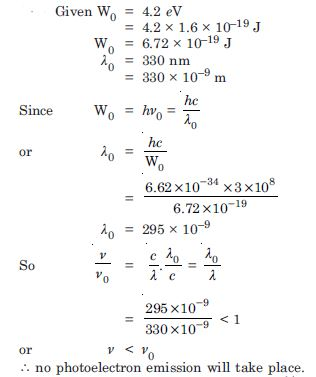##### Question 9:

Light of frequency 7.21 × 1014 Hz is incident on a metal surface. Electrons with a maximum speed of 6.0 × 105m/s are ejected from the surface. What is the threshold frequency for photoemission of electrons ?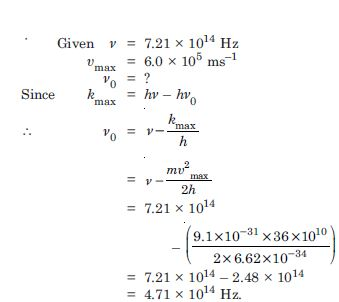##### Question 10:

Light of wavelength 488 nm is produced by an argon laser which is used in the photoelectric effect. When light from this spectral line is incident on the emitter, the stopping (cut-off) potential of photoelectrons is 0.38 V. Find the work function of the material from which the emitter is made.

Given $\mathrm{\lambda }$ = 488 nm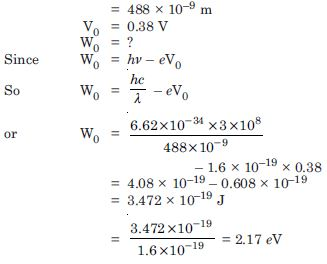Sub-Topic - Wave Nature of Matter
##### Question 1:

Calculate the momentum, and de-Broglie wavelength of the electrons accelerated through a potential difference of 56 V.

Given V = 56 volt
p = ?, $\mathrm{\lambda }$ = ?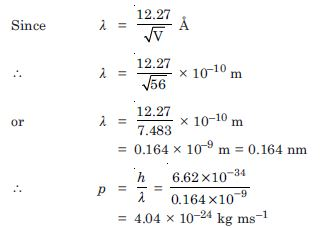##### Question 2:

What is the momentum, (b) speed and de-Broglie wavelength of an electron with kinetic energy of 120 eV ?

Given E = 120 eV = 120 × 1.6 × 10–19 J =
192 × 10–19 J
p = ?, υ = ?,
$\mathrm{\lambda }$ = ?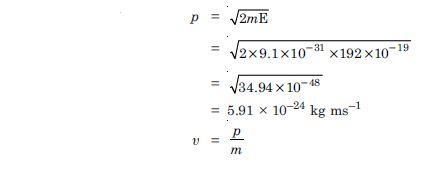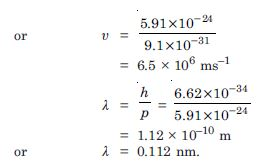##### Question 3:

The wavelength of light from the spectral emission line of sodium is 589 nm. Find the kinetic energy at which an electron and a neutron, would have the same de-Broglie wavelength.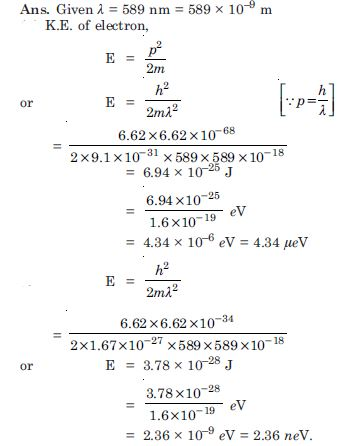##### Question 4:

What is the de-Broglie wavelength of
a bullet of mass 0.040 kg travelling at the speed of 1.0 km/s,
a ball of mass 0.060 kg moving at a speed of 1.0 m/s and
a dust particle of mass 1.0 × 10–9 kg drifting with a speed of 2.2 m/s ?##### Question 5:

An electron and a photon each have a wavelength of 1.00 nm. Find : their momenta the energy of the photon and the kinetic energy of electron.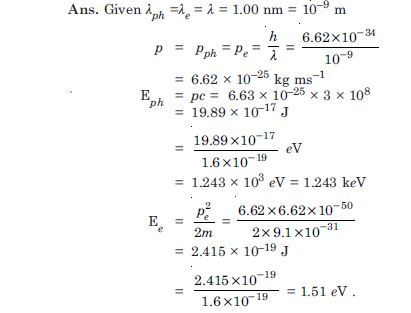##### Question 6: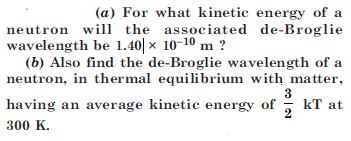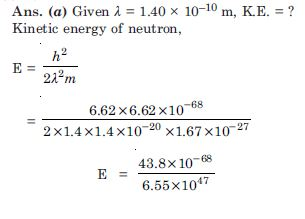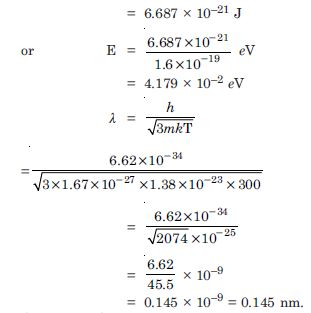##### Question 7:

Show that the wavelength of electromagnetic radiation is equal to the de-Broglie wavelength of its quantum (Photon).

Since de-Broglie wavelength of a photon is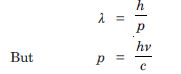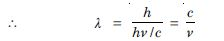Which is the wavelength of electromagnetic radiations.

##### Question 8:

What is the de-Broglie wavelength of a nitrogen molecule in air at 300 K ? Assume that the molecule is moving with the root-meansquare speed of molecules at this temperature. (Atomic mass of nitrogen = 14.0076 u).